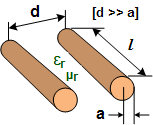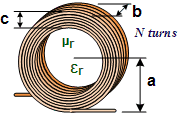About RF CafeCopyright: 1996 - 2024Webmaster:    Kirt Blattenberger,     BSEE - KB3UON RF Cafe began life in 1996 as "RF Tools" in an AOL screen name web space totaling 2 MB. Its primary purpose was to provide me with ready access to commonly needed formulas and reference material while performing my work as an RF system and circuit design engineer. The World Wide Web (Internet) was largely an unknown entity at the time and bandwidth was a scarce commodity. Dial-up modems blazed along at 14.4 kbps while typing up your telephone line, and a nice lady's voice announced "You've Got Mail" when a new message arrived... All trademarks, copyrights, patents, and other rights of ownership to images and text used on the RF Cafe website are hereby acknowledged. My Hobby Website: AirplanesAndRockets.com# Inductors & Inductance Calculations

Inductors are passive devices used in electronic circuits to store energy in the form of a magnetic field. They are the compliment of capacitors, which store energy in the form of an electric field. An ideal inductor is the equivalent of a short circuit (0 ohms) for direct currents (DC), and presents an opposing force (reactance) to alternating currents (AC) that depends on the frequency of the current. The reactance (opposition to current flow) of an inductor is proportional to the frequency of the current flowing through it. Inductors are sometimes referred to as "coils" because most inductors are physically constructed of coiled sections of wire.

The property of inductance that opposes a change in current flow is exploited for the purpose of preventing signals with a higher frequency component from passing while allowing signals of lower frequency components to pass. This is why inductors are sometimes referred to as "chokes," since they effectively choke off higher frequencies. A common application of a choke is in a radio amplifier biasing circuit where the collector of a transistor needs to be supplied with a DC voltage without allowing the RF (radio frequency) signal from conducting back into the DC supply.When used in series (left drawing) or parallel (right drawing) with its circuit compliment, a capacitor, the inductor-capacitor combination forms a circuit that resonates at a particular frequency that depends on the values of each component. In the series circuit, the impedance to current flow at the resonant frequency is zero with ideal components. In the parallel circuit (right), impedance to current flow is infinite with ideal components.Real-world inductors made of physical components exhibit more than just a pure inductance when present in an AC circuit. A common circuit simulator model is shown to the left. It includes the actual ideal inductor with a parallel resistive component that responds to alternating current. The DC resistive component is in series with the ideal inductor, and a capacitor is connected across the entire assembly and represents the capacitance present due to the proximity of the coil windings. SPICE-type simulators use this or an even more sophisticated model to facilitate more accurate calculations over a wide range of frequencies.

Related Pages on RF Cafe
- Inductors & Inductance Calculations
- Inductance Conversions
- Standard Inductor Values
- Inductor Vendors

The HamWaves.com website has a very sophisticated calculator for coil inductance that allows you to en9ter the conductor diameter.

Equations (formulas) for combining inductors in series and parallel are given below. Additional equations are given for inductors of various configurations.

## Series-Connected InductorsTotal inductance of series-connected inductors is equal to the sum of the individual inductances. Keep units constant.

## Closely Wound Toroid

Rectangular Cross-Section## Coaxial Cable Inductance## Straight Wire InductanceThese equations apply for when the length of the wire is much longer than the wire diameter (look up wire diameter here). The ARRL Handbook presents the equation for units of inches and µF:

For lower frequencies - up through about VHF, use this formula:Above VHF, skin effect causes the ¾ in the top equation to approach unity (1), so use this equation:## Straight Wire Parallel to Ground Plane w/One End GroundedThe ARRL Handbook presents this equation for a straight wire suspended above a ground plane, with one end grounded to the plane:

a = wire radius,  l = wire length parallel to ground plane
h = height of wire above ground plane to bottom of wire

## Parallel Line Inductance## Multi-Layer Air-Core InductanceWheeler's Formula:## Parallel-Connected InductorsTotal inductance of parallel-connected inductors is equal to the reciprocal of the sum of the reciprocals of the individual inductances. Keep units constant.

## Inductance Formula Constants and Variables

The following physical constants and mechanical dimensional variables apply to equations on this page. Units for equations are shown inside brackets at the end of equations; e.g.,means lengths are in inches and inductance is in Henries. If no units are indicated, then any may be used so long as they are consistent across all entities; i.e., all meters, all µH, etc.

C = Capacitance
L = Inductance
N = Number of turns
W = Energy
εr = Relative permittivity (dimensionless)
ε0 = 8.85 x 10-12 F/m (permittivity of free space)
µr = Relative permeability (dimensionless)
µ0 = 4π x 10-7 H/m (permeability of free space)

1 meter = 3.2808 feet  <—>  1 foot = 0.3048 meters
1 mm = 0.03937 inches  <—>  1 inch = 25.4 mm

Also, dots (not to be confused with decimal points) are used to indicate multiplication in order to avoid ambiguity.

## Inductive ReactanceInductive reactance (XL, in Ω) is proportional to the frequency (ω, in radians/sec, or f, in Hz) and inductance (L, in Henries). Pure inductance has a phase angle of 90° (voltage leads current with a phase angle of 90°).

## Energy Stored in an InductorEnergy (W, in Joules) stored in an inductor is half the product of the inductance (L, in Henries) and the current (I, in amp) through the device.

## Voltage Across an InductorThe inductor's property of opposing a change in current flow causes a counter EMF (voltage) to form across its terminals opposite in polarity to the applied voltage.

## Quality Factor of InductorQuality factor is the dimensionless ratio of reactance to resistance in an inductor.

## Single-Layer Round Coil InductanceWheeler's Formula for d >> a:In general for a = wire radius:Note: If lead lengths are significant, use the straight wire calculation to add that inductance.

## Finding the Equivalent "RQ"Since the "Q" of an inductor is the ratio of the reactive component to the resistive component, an equivalent circuit can be defined with a resistor in parallel with the inductor. This equation is valid only at a single frequency, "f," and must be calculated for each frequency of interest.Please Support RF Cafe by purchasing my  ridiculously low−priced products, all of which I created. These Are Available for Free